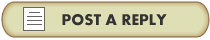Home UFO Topics UFO Photographs UFO Cases Sighting Reports Report a UFO Sighting

UFO Evidence Forums : Debate - Are UFOs Real?
 User not logged in - Login or Register

Replies 11 - 15 (out of 15 total)
 equals 86 7/7/2004 6:12:53 PM kamarinas @ uh.edu uh+1=edu=29 uh*3=kamarinas=87 kevin-uh=32 arquiza-uh=64=(kevin-uh)*2 87-64=23 marinas-uh=46 (kevin-uh)*(marinas-uh)/(arquiza-uh)=23 (kevin-uh)+(marinas-uh)=78 (arquiza-uh)*(marinas-uh)/(kevin-uh)=92 29 87 32 64 46 23 78 92 kevin-uh*2=3 arquiza-uh*2=35 marinas-uh*2=17 (3*35)+17 + (3*17)+35 + (17*35)+3 = 806 (August 6 or 86) ((3*35)+17) * ((3*17)+35) * ((17*35)+3) = 6274216 6+2+7+4+2+1+6=28 6*2*7*4*2*1*6=4032 4032/28=144 it's another one of those 144's (this is the third one i found)
 equals 86 7/12/2004 6:07:44 PM there is a new correction to be made from the 8th post (the 2nd from the last post on the first page of 10 posts): // 48/486=a repeating decimal with all digits in decreasing order except 1 86/2=43 1+8+4+9=22 add 1 to get 23 1*8*4*9=288 add 1 to get 17 squared I am 17 but I am the 86 1986+17=2003 add one to get 2004 // the correction: 48/486=a repeating decimal with all digits in decreasing order except 1 86/2=43 >> 43^2=1849 1+8+4+9=22 add 1 to get 23 1*8*4*9=288 add 1 to get 17 squared I am 17 >> 288/2=144 (yet another 144!) but I am the 86 1986+17=2003 add one to get 2004 1986+144=2130 2*1*3=2+1+3=6 2130*6=12780 1+2+7+8=20 1*2*7*8=112 refer to the many previous posts for details and to discover further relevance.
 equals 86 7/12/2004 6:23:11 PM in addition to the previous post: 1278/86=14.860465116279069767441860465116 14.860465116279069767441860465116 is a repeating decimal 14. 860465116279069767441 860465116279069767441 8+6+0+4+6+5+1+1+6+2+7+9+0+6+9+7+6+7+4+4+1=99 8*6*4*6*5*1*1*6*2*7*9*6*9*7*6*7*4*4*1=1106127912960 9+9=18 1+1+0+6+1+2+7+9+1+2+9+6+0=45 1+8=4+5 18*45=810 1106127912960/810=1365590016 1+3+6+5+5+9+0+0+1+6=36 (136559/16)/36=237.08159722222222222222222222222... 239th day of year = my b-day 239-237.08159722222222222222222222222...=1.9184027777777777777777777777777... 9*8*1*4=288 27 followed by and infinity of 7's
 equals 86 7/12/2004 6:34:55 PM in addition to the yet previous post: >> (136559/16)/36=237.08159722222222222222222222222... 16*36=376 576/2=288 288/2=144 again!
 equals 86 7/12/2004 6:36:41 PM in post 14, i made a mistake... interactively, you can find out what this error is.

Replies 11 - 15 (out of 15 total)